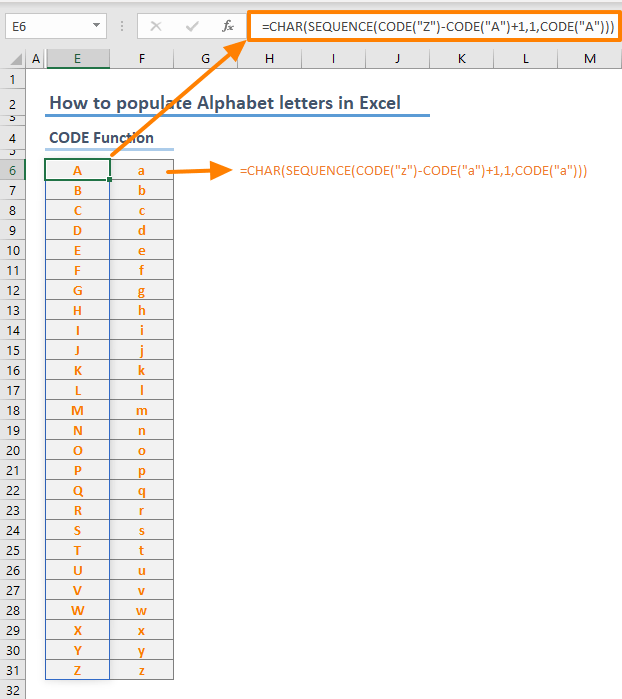You can populate numbers, names of months or weekdays easily by dragging your mouse. However, you can’t populate letters in the same fashion. In this guide, we're going to show you how to populate Alphabet letters in Excel using three different methods.

## Introduction

Let us first take a look at how character sets work in Excel. There are two built-in functions which are related to converting numbers into characters:

We can use these functions to convert numbers and letters from one another to populate letters in Excel.

Although Windows and Mac systems use different character sets, they share same numbering for letters. For a detailed list see:

## Simpler Method

Uppercase and lowercase letters in ASCII character set reside between the numbers 65-90 and 97-122, respectively. 65 = A, 66 = B, 90 = Z, 106 = j, etc. Using character conversion formulas like CHAR, we can convert this numbers to corresponding letters.

Syntax

CHAR(<number>)

Example

=CHAR(121) returns “y”

The challenge for this approach is generating numbers for the CHAR function. As explained in the previous section, you need numbers between 65 and 90 or 97 and 122 for letters. For this, you can use the SEQUENCE function at this step. The SEQUENCE function can generate an array of sequential numbers automatically.

All you need to do is to supply how many numbers you need, and which number to start with.

Syntax

SEQUENCE(rows,[columns],[start],[step])

Example

=SEQUENCE(26,1,65) returns an array of numbers between 65 and 90

The function above returns numbers into 26 rows and single column. If you want to generate numbers or letters like in our example, you can use a formula like =SEQUENCE(1,26,65).

Let’s combine both functions to create formula for populating letters of the alphabet.

Syntax

=CHAR(SEQUENCE(26,1,<number where letters start>))

Example

Uppercase letters: =CHAR(SEQUENCE(26,1,65))Lowercase letters: =CHAR(SEQUENCE(26,1,97))## Using the CODE function for populating letters of the alphabet

Excel's has the CODE function works in opposite way of the CHAR. The CODE function simply returns the corresponding number of the given character in a character set.

Syntax

CODE(text)

Example

CODE(“c”) returns 99

As a result, the CODE function with the character can be replaced with the number itself. The following is an example for using this formula.

Syntax

=CHAR(SEQUENCE(CODE(<last character>)-CODE(<first character> )+1,1,CODE(<first character>)))

Example

=CHAR(SEQUENCE(CODE("Z")-CODE("A")+1,1,CODE("A")))## Dynamic character interval

Using the CODE function, we can improve the model by following a more dynamic approach. We can replace the hard coded letters like “A” or “Z” with cell references containing these characters. This approach allows us to change the start and end characters of alphabet letters without updating the formula.

Syntax

=CHAR(SEQUENCE(CODE(<the cell contains the last character>)-CODE(<the cell contains the first character> )+1,1,CODE(<the cell contains the first character>)))

Example

=CHAR(SEQUENCE(CODE(I7)-CODE(I6)+1,1,CODE(I6)))## Using LET function to populate Alphabet letters

This is an optional step for populating alphabet letters in Excel. The relatively new LET function allows you to create named ranges inside the context of its own formula.

The syntax of the LET function contains the actual formula which using determined name-value pairs.

Syntax of the LET function

LET(name1, name_value1, [name2], [name_value2], …, calculation)

Syntax of our model with the LET function

=LET(Start,CODE(<the cell contains the first character>),Finish,CODE(<the cell contains the last character>),CHAR(SEQUENCE(Start-Finish+1,1,Finish)))

Example

=LET(Start,CODE(M7),Finish,CODE(M6),CHAR(SEQUENCE(Start-Finish+1,1,Finish)))

We defined named ranges for Start and Finish as CODE(M7) and CODE(M6) respectively. Each name is replaced with the corresponding CODE function block.